Updating search results...

# 812 Results

View
Selected filters: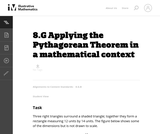Unrestricted Use
CC BY
Rating
0.0 stars

This task requires students to apply the Pythagorean Theorem.

Subject:
Mathematics
Geometry
Material Type:
Activity/Lab
Provider:
Illustrative Mathematics
Provider Set:
Illustrative Mathematics
Author:
Illustrative Mathematics
05/01/2012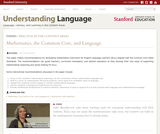Some Rights Reserved
Rating
0.0 stars

This paper makes recommendations for developing mathematics instruction for English Language Learners (ELLs) aligned with the Common Core State Standards. The recommendations can guide teachers, curriculum developers, and teacher educators as they develop their own ways of supporting mathematical reasoning and sense-making for ELLs.Some instructional recommendations discussed in the paper include: Focus on ELL students' mathematical reasoning, not the correctness of their mathematical language use. Shift to a focus on mathematical discourse practices; move away from simplified views of language. Support ELL students as they engage in complex mathematical language. Use ELL students' language and experiences as resources. Provide professional development to enhance teachers' awareness of ways to support ELs as they develop both language and mathematical knowledge.

Subject:
Language Education (ESL)
Material Type:
Teaching/Learning Strategy
Provider:
Stanford University School of Education
Provider Set:
Understanding Language
Author:
Judit Moschkovich
03/02/2012Unrestricted Use
CC BY
Rating
5.0 stars

Mathematically Productive Instructional Routines (MPIR) are short (10ish minutes), daily exercises aimed at building number sense. These six different MPIR are part of the Mathematically Productive Instructional Routines collection from the Washington Office of Public Instruction and the Washington Association of Educational Service Districts.

Subject:
Mathematics
Material Type:
Teaching/Learning Strategy
Author:
Barbara Soots
Washington OSPI Mathematics Department
Washington OSPI OER Project
04/05/2021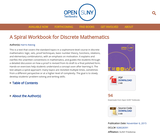Conditional Remix & Share Permitted
CC BY-NC-SA
Rating
0.0 stars

This is a text that covers the standard topics in a sophomore-level course in discrete mathematics: logic, sets, proof techniques, basic number theory, functions, relations, and elementary combinatorics, with an emphasis on motivation. It explains and clarifies the unwritten conventions in mathematics, and guides the students through a detailed discussion on how a proof is revised from its draft to a final polished form. Hands-on exercises help students understand a concept soon after learning it. The text adopts a spiral approach: many topics are revisited multiple times, sometimes from a different perspective or at a higher level of complexity. The goal is to slowly develop students’ problem-solving and writing skills.

Subject:
Computer Science
Mathematics
Functions
Numbers and Operations
Material Type:
Textbook
Provider:
State University of New York
Provider Set:
Milne Open Textbooks
Author:
Harris Kwong
11/06/2015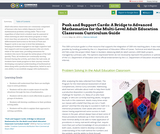Conditional Remix & Share Permitted
CC BY-NC-SA
Rating
5.0 stars

Adult education classrooms are commonly comprised of learners who have widely disparate levels of mathematical problem-solving skills. This is true regardless of what level a student may be assessed at when entering an adult education program or what level class they are placed in. Providing students with differentiated instruction in the form of Push and Support cards is one way to level this imbalance, keeping all students engaged in one high-cognitive task that supports and encourages learners who are stuck, while at the same time, providing extensions for students who move through the initial phase of the task quickly. Thus, all
students are continually moving forward during the activity, and when the task ends, all students have made progress in their journey towards developing conceptual understanding of mathematical ideas along with a productive disposition, belief in one’s own ability to successfully engage with mathematics.

Subject:
Mathematics
Material Type:
Lesson Plan
Module
Teaching/Learning Strategy
Author:
Patricia Helmuth
05/24/2018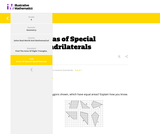Conditional Remix & Share Permitted
CC BY-NC-SA
Rating
0.0 stars

This task asks students to identify which of the six polygons have the same area. Students may complete the task using a variety of techniques including decomposing shapes, using transformations (rotations, reflections, translations) to move one or more parts of the figure to another part to more easily calculate the area, enclosing the polygon inside a larger rectangle and then subtract the areas of the "extra" pieces, etc.

Subject:
Mathematics
Material Type:
Activity/Lab
Diagram/Illustration
Lesson Plan
Provider:
Illustrative Mathematics
05/08/2020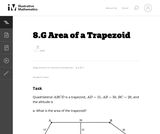Unrestricted Use
CC BY
Rating
0.0 stars

This is a task from the Illustrative Mathematics website that is one part of a complete illustration of the standard to which it is aligned. Each task has at least one solution and some commentary that addresses important asects of the task and its potential use. Here are the first few lines of the commentary for this task: Quadrilateral $ABCD$ is a trapezoid, $AD = 15$, $AB = 50$, $BC = 20$, and the altitude is 12. What is the area of the trapezoid?...

Subject:
Mathematics
Material Type:
Activity/Lab
Provider:
Illustrative Mathematics
Provider Set:
Illustrative Mathematics
Author:
Illustrative Mathematics
03/17/2013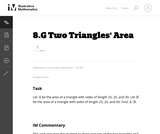Unrestricted Use
CC BY
Rating
0.0 stars

This is a task from the Illustrative Mathematics website that is one part of a complete illustration of the standard to which it is aligned. Each task has at least one solution and some commentary that addresses important asects of the task and its potential use. Here are the first few lines of the commentary for this task: Let $A$ be the area of a triangle with sides of length 25, 25, and 30. Let $B$ be the area of a triangle with sides of length 25, 25, and 40. Find A/B... Subject: Mathematics Material Type: Activity/Lab Provider: Illustrative Mathematics Provider Set: Illustrative Mathematics Author: Illustrative Mathematics Date Added: 05/29/2013Unrestricted Use CC BY Rating 0.0 stars This is a task from the Illustrative Mathematics website that is one part of a complete illustration of the standard to which it is aligned. Each task has at least one solution and some commentary that addresses important asects of the task and its potential use. Here are the first few lines of the commentary for this task: During the 2005 Divisional Playoff game between The Denver Broncos and The New England Patriots, Bronco player Champ Bailey intercepted Tom Brady aroun... Subject: Mathematics Material Type: Activity/Lab Provider: Illustrative Mathematics Provider Set: Illustrative Mathematics Author: Illustrative Mathematics Date Added: 03/17/2013Unrestricted Use CC BY Rating 0.0 stars This is a task from the Illustrative Mathematics website that is one part of a complete illustration of the standard to which it is aligned. Each task has at least one solution and some commentary that addresses important aspects of the task and its potential use. Subject: Geometry Material Type: Activity/Lab Provider: Illustrative Mathematics Provider Set: Illustrative Mathematics Author: Illustrative Mathematics Date Added: 08/06/2015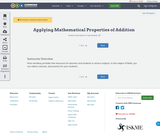Unrestricted Use CC BY Rating 0.0 stars Khan Aacdemy provides free resources for teachers and students in various subjects. In the subject of Math, you can videos; tutorials, and practice for your students. Subject: Mathematics Material Type: Lesson Author: Jodi Barnes Date Added: 08/18/2022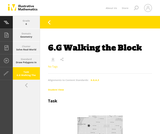Unrestricted Use CC BY Rating 0.0 stars This is a task from the Illustrative Mathematics website that is one part of a complete illustration of the standard to which it is aligned. Each task has at least one solution and some commentary that addresses important aspects of the task and its potential use. Subject: Geometry Material Type: Activity/Lab Provider: Illustrative Mathematics Provider Set: Illustrative Mathematics Author: Illustrative Mathematics Date Added: 08/06/2015Conditional Remix & Share Permitted CC BY-NC Rating 0.0 stars Students review the ways classroom habits and routines can strengthen their mathematical character. Students learn what a Gallery is and how to choose a Gallery problem to work on. They then choose one of three Gallery problems that introduce the unit’s technology resources. The three Gallery problems combine working with expressions with the resources available with this unit.Key ConceptsUnderstand that a Gallery gives students a choice of several problems. Understand what to consider when choosing a problem. Know how to work on a Gallery problem and how to present work on gallery problems.Goals and Learning ObjectivesKnow how to choose a problem from a Gallery. Subject: Mathematics Material Type: Lesson Plan Provider: Pearson Date Added: 09/21/2015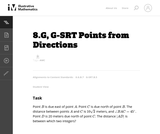Unrestricted Use CC BY Rating 0.0 stars This is a task from the Illustrative Mathematics website that is one part of a complete illustration of the standard to which it is aligned. Each task has at least one solution and some commentary that addresses important asects of the task and its potential use. Here are the first few lines of the commentary for this task: PointB$is due east of point$A$. Point$C$is due north of point$B$. The distance between points$A$and$C$is$10\sqrt 2$meters, and$\angle BAC...

Subject:
Mathematics
Material Type:
Activity/Lab
Provider:
Illustrative Mathematics
Provider Set:
Illustrative Mathematics
Author:
Illustrative Mathematics
03/29/2013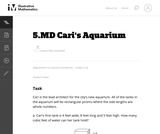Unrestricted Use
CC BY
Rating
0.0 stars

This is a task from the Illustrative Mathematics website that is one part of a complete illustration of the standard to which it is aligned. Each task has at least one solution and some commentary that addresses important asects of the task and its potential use. Here are the first few lines of the commentary for this task: Cari is the lead architect for the city’s new aquarium. All of the tanks in the aquarium will be rectangular prisms where the side lengths are whole nu...

Subject:
Mathematics
Material Type:
Activity/Lab
Provider:
Illustrative Mathematics
Provider Set:
Illustrative Mathematics
Author:
Illustrative Mathematics
05/13/2013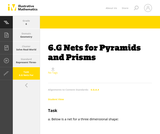Unrestricted Use
CC BY
Rating
0.0 stars

This is a task from the Illustrative Mathematics website that is one part of a complete illustration of the standard to which it is aligned. Each task has at least one solution and some commentary that addresses important aspects of the task and its potential use.

Subject:
Geometry
Material Type:
Activity/Lab
Provider:
Illustrative Mathematics
Provider Set:
Illustrative Mathematics
Author:
Illustrative Mathematics
08/06/2015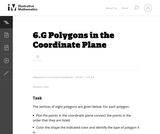Unrestricted Use
CC BY
Rating
0.0 stars

This is a task from the Illustrative Mathematics website that is one part of a complete illustration of the standard to which it is aligned. Each task has at least one solution and some commentary that addresses important asects of the task and its potential use. Here are the first few lines of the commentary for this task: The vertices of eight polygons are given below. For each polygon: * Plot the points in the coordinate plane connect the points in the order that they a...

Subject:
Mathematics
Material Type:
Activity/Lab
Provider:
Illustrative Mathematics
Provider Set:
Illustrative Mathematics
Author:
Illustrative Mathematics
09/08/2013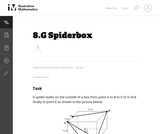Unrestricted Use
CC BY
Rating
0.0 stars

This is a task from the Illustrative Mathematics website that is one part of a complete illustration of the standard to which it is aligned. Each task has at least one solution and some commentary that addresses important asects of the task and its potential use. Here are the first few lines of the commentary for this task: A spider walks on the outside of a box from point A to B to C to D and finally to point E as shown in the picture below. Draw a net of the box and map ...

Subject:
Mathematics
Material Type:
Activity/Lab
Provider:
Illustrative Mathematics
Provider Set:
Illustrative Mathematics
Author:
Illustrative Mathematics
11/25/2013Unrestricted Use
CC BY
Rating
0.0 stars

This is a task from the Illustrative Mathematics website that is one part of a complete illustration of the standard to which it is aligned. Each task has at least one solution and some commentary that addresses important asects of the task and its potential use. Here are the first few lines of the commentary for this task: At the beginning of the month, Evan had \24 in his account at the school bookstore. Use a variable to represent the unknown quantity in each transacti... Subject: Mathematics Material Type: Activity/Lab Provider: Illustrative Mathematics Provider Set: Illustrative Mathematics Author: Illustrative Mathematics Date Added: 05/13/2013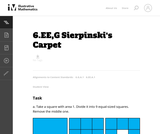Unrestricted Use CC BY Rating 0.0 stars This is a task from the Illustrative Mathematics website that is one part of a complete illustration of the standard to which it is aligned. Each task has at least one solution and some commentary that addresses important asects of the task and its potential use. Here are the first few lines of the commentary for this task: Take a square with area 1. Divide it into 9 equal-sized squares. Remove the middle one. What is the area of the figure now? Take the remaining 8 square... Subject: Mathematics Material Type: Activity/Lab Provider: Illustrative Mathematics Provider Set: Illustrative Mathematics Author: Illustrative Mathematics Date Added: 08/06/2013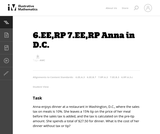Unrestricted Use CC BY Rating 0.0 stars This is a task from the Illustrative Mathematics website that is one part of a complete illustration of the standard to which it is aligned. Each task has at least one solution and some commentary that addresses important asects of the task and its potential use. Here are the first few lines of the commentary for this task: Anna enjoys dinner at a restaurant in Washington, D.C., where the sales tax on meals is 10%. She leaves a 15% tip on the price of her meal before the s... Subject: Mathematics Material Type: Activity/Lab Provider: Illustrative Mathematics Provider Set: Illustrative Mathematics Author: Illustrative Mathematics Date Added: 03/17/2013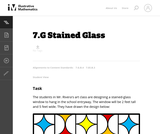Unrestricted Use CC BY Rating 0.0 stars This is a task from the Illustrative Mathematics website that is one part of a complete illustration of the standard to which it is aligned. Each task has at least one solution and some commentary that addresses important asects of the task and its potential use. Here are the first few lines of the commentary for this task: The students in Mr. Rivera's art class are designing a stained-glass window to hang in the school entryway. The window will be 2 feet tall and 5 feet w... Subject: Mathematics Material Type: Activity/Lab Provider: Illustrative Mathematics Provider Set: Illustrative Mathematics Author: Illustrative Mathematics Date Added: 08/19/2013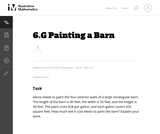Unrestricted Use CC BY Rating 0.0 stars The purpose of this task is to provide students an opportunity to use mathematics addressed in different standards in the same problem. Subject: Mathematics Geometry Material Type: Activity/Lab Provider: Illustrative Mathematics Provider Set: Illustrative Mathematics Author: Illustrative Mathematics Date Added: 05/01/2012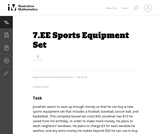Unrestricted Use CC BY Rating 0.0 stars The purpose of this task is to present students with a context that can naturally be represented with an inequality and to explore the relationship between the context and the mathematical representation of that context; thus, this is an intended as an instructional task. Subject: Mathematics Material Type: Activity/Lab Provider: Illustrative Mathematics Provider Set: Illustrative Mathematics Author: Illustrative Mathematics Date Added: 08/10/2012Unrestricted Use CC BY Rating 0.0 stars This purpose of this task is to help students see two different ways to look at percentages both as a decrease and an increase of an original amount. In addition, students have to turn a verbal description of several operations into mathematical symbols. Subject: Mathematics Algebra Material Type: Activity/Lab Provider: Illustrative Mathematics Provider Set: Illustrative Mathematics Author: Illustrative Mathematics Date Added: 05/01/2012Unrestricted Use CC BY Rating 0.0 stars This is a task from the Illustrative Mathematics website that is one part of a complete illustration of the standard to which it is aligned. Each task has at least one solution and some commentary that addresses important asects of the task and its potential use. Here are the first few lines of the commentary for this task: The taxi fare in Gotham City is \2.40 for the first $\frac{1}{2}$ mile and additional mileage charged at the rate \\$0.20 for each additional 0.1 mile....

Subject:
Mathematics
Material Type:
Activity/Lab
Provider:
Illustrative Mathematics
Provider Set:
Illustrative Mathematics
Author:
Illustrative Mathematics
03/17/2013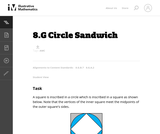Unrestricted Use
CC BY
Rating
0.0 stars

This is a task from the Illustrative Mathematics website that is one part of a complete illustration of the standard to which it is aligned. Each task has at least one solution and some commentary that addresses important asects of the task and its potential use. Here are the first few lines of the commentary for this task: A square is inscribed in a circle which is inscribed in a square as shown below. Note that the vertices of the inner square meet the midpoints of the o...

Subject:
Mathematics
Material Type:
Activity/Lab
Provider:
Illustrative Mathematics
Provider Set:
Illustrative Mathematics
Author:
Illustrative Mathematics
09/08/2013Only Sharing Permitted
CC BY-NC-ND
Rating
4.0 stars

This lesson unit is intended to help you assess how well students are able to use geometric properties to solve problems. In particular, it will support you in identifying and helping students who have the following difficulties: Solving problems relating to using the measures of the interior angles of polygons; and solving problems relating to using the measures of the exterior angles of polygons.

Subject:
Geometry
Material Type:
Assessment
Lesson Plan
Provider:
Shell Center for Mathematical Education
Provider Set:
Mathematics Assessment Project (MAP)
04/26/2013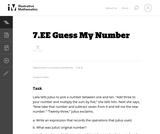Unrestricted Use
CC BY
Rating
0.0 stars

This problem asks the students to represent a sequence of operations using an expression and then to write and solve simple equations. The problem is posed as a game and allows the students to visualize mathematical operations. It would make sense to actually play a similar game in pairs first and then ask the students to record the operations to figure out each other's numbers.

Subject:
Mathematics
Algebra
Material Type:
Activity/Lab
Provider:
Illustrative Mathematics
Provider Set:
Illustrative Mathematics
Author:
Illustrative Mathematics
08/07/2012Unrestricted Use
CC BY
Rating
0.0 stars

This is a task from the Illustrative Mathematics website that is one part of a complete illustration of the standard to which it is aligned. Each task has at least one solution and some commentary that addresses important asects of the task and its potential use. Here are the first few lines of the commentary for this task: Below is a table showing the number of hits and the number of times at bat for two Major League Baseball players during two different seasons: SeasonDe...

Subject:
Mathematics
Material Type:
Activity/Lab
Provider:
Illustrative Mathematics
Provider Set:
Illustrative Mathematics
Author:
Illustrative Mathematics
08/10/2013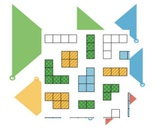Conditional Remix & Share Permitted
CC BY-NC
Rating
0.0 stars

Download GeoGebra Illustrative Mathematics LMS course files. All of GeoGebra's Illustrative Mathematics apps have been placed via links into Moodle courses - each unit is one course. There are twenty save middles school courses and more for high school (the list is growing.) These course files can then be uploaded into Moodle, Schoology, Canvas or D2L.

Subject:
Mathematics
Material Type:
Full Course
Author:
Dan MGuire
Diana Fisher Ph.D.
04/07/2021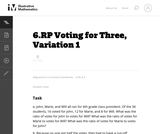Unrestricted Use
CC BY
Rating
0.0 stars

This problem is the fifth in a series of seven about ratios. In the first problem students define the simple ratios that exist among the three candidates. It opens an opportunity to introduce unit rates. The subsequent problems are more complex. In the second problem, students apply their understanding of ratios to combine two pools of voters to determine a new ratio. In the third problem, students apply a known ratio to a new, larger pool of voters to determine the number of votes that would be garnered.

Subject:
Mathematics
Material Type:
Activity/Lab
Provider:
Illustrative Mathematics
Provider Set:
Illustrative Mathematics
Author:
Illustrative Mathematics
05/01/2012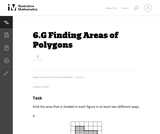Unrestricted Use
CC BY
Rating
0.0 stars

The purpose of this task is to have students work on a sequence of area problems that shows the advantage of increasingly abstract strategies in preparation for developing general area formulas for parallelograms and triangles.

Subject:
Mathematics
Geometry
Material Type:
Activity/Lab
Provider:
Illustrative Mathematics
Provider Set:
Illustrative Mathematics
Author:
Illustrative Mathematics
05/01/2012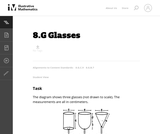Unrestricted Use
CC BY
Rating
0.0 stars

This task gives students an opportunity to work with volumes of cylinders, spheres and cones. Notice that the insight required increases as you move across the three glasses, from a simple application of the formula for the volume of a cylinder, to a situation requiring decomposition of the volume into two pieces, to one where a height must be calculated using the Pythagorean theorem.

Subject:
Mathematics
Geometry
Material Type:
Activity/Lab
Provider:
Illustrative Mathematics
Provider Set:
Illustrative Mathematics
Author:
Illustrative Mathematics
05/01/2012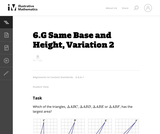Unrestricted Use
CC BY
Rating
0.0 stars

This is the second version of a task asking students to find the areas of triangles that have the same base and height. This presentation is more abstract as students are not using physical models.

Subject:
Mathematics
Geometry
Material Type:
Activity/Lab
Provider:
Illustrative Mathematics
Provider Set:
Illustrative Mathematics
Author:
Illustrative Mathematics
05/01/2012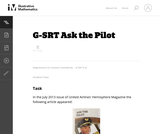Unrestricted Use
CC BY
Rating
0.0 stars

This is a task from the Illustrative Mathematics website that is one part of a complete illustration of the standard to which it is aligned. Each task has at least one solution and some commentary that addresses important asects of the task and its potential use. Here are the first few lines of the commentary for this task: In the July 2013 issue of United Airlines' Hemisphere Magazine the following article appeared: Write down an equation that describes Captain Bowers' me...

Subject:
Mathematics
Material Type:
Activity/Lab
Provider:
Illustrative Mathematics
Provider Set:
Illustrative Mathematics
Author:
Illustrative Mathematics
10/13/2013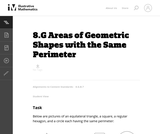Unrestricted Use
CC BY
Rating
0.0 stars

This problem is part of a very rich tradition of problems looking to maximize the area enclosed by a shape with fixed perimeter. Only three shapes are considered here because the problem is difficult for more irregular shapes.

Subject:
Mathematics
Geometry
Material Type:
Activity/Lab
Provider:
Illustrative Mathematics
Provider Set:
Illustrative Mathematics
Author:
Illustrative Mathematics
01/20/2013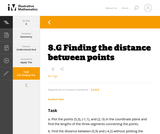Unrestricted Use
CC BY
Rating
0.0 stars

This is a task from the Illustrative Mathematics website that is one part of a complete illustration of the standard to which it is aligned. Each task has at least one solution and some commentary that addresses important aspects of the task and its potential use.

Subject:
Geometry
Material Type:
Activity/Lab
Provider:
Illustrative Mathematics
Provider Set:
Illustrative Mathematics
Author:
Illustrative Mathematics
08/06/2015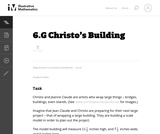Unrestricted Use
CC BY
Rating
0.0 stars

This task is primarily about volume and surface area, although it also gives students an early look at converting between measurements in scale models and the real objects they correspond to.

Subject:
Mathematics
Geometry
Material Type:
Activity/Lab
Provider:
Illustrative Mathematics
Provider Set:
Illustrative Mathematics
Author:
Illustrative Mathematics
05/01/2012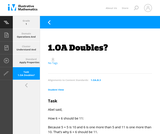Unrestricted Use
CC BY
Rating
0.0 stars

This is a task from the Illustrative Mathematics website that is one part of a complete illustration of the standard to which it is aligned. Each task has at least one solution and some commentary that addresses important aspects of the task and its potential use.

Subject:
Algebra
Material Type:
Activity/Lab
Provider:
Illustrative Mathematics
Provider Set:
Illustrative Mathematics
Author:
Illustrative Mathematics